# Mathematics Paper 2 Questions and Answers - Form 4 Term 1 Opener Exams 2021

FORM 4 TERM 1
OPENER EXAMS

MATHEMATICS PAPER 2
TIME: 2½ HRS.

INSTRUCTION TO STUDENTS:

• This paper consists of two Sections; Section I and Section II.
• Answer ALL the questions in Section I and only five questions from Section II.
• Show all the steps in your calculation, giving your answer at each stage in the spaces provided below each question.
• Marks may be given for correct working even if the answer is wrong.
• KNEC Mathematical tables may be used, except where stated otherwise.
• Candidates should answer the questions in English.

SECTION I

1. Use logarithms to evaluate.(3 marks)
4.497 x √0.3673
1- cos 81.53°
2. The sum of the fifth and sixth term of an AP is 30.If the third term is 5.Find the first term.(3marks)
3. Make K the subject of the formula and simplify.(3marks)
t= 2y+1
√2ky+k
4. Solve for x
2-(log x)2=3 log x (3marks)
5. The sides of a triangle were measured and recorded as 4cm,6.2cm and 9.50cm.Calculate the percentage error in its perimeter correct to 2 decimal places. (3marks)
6. The figure below shows a triangle PQR in which PQ=8cm and angle QPR=1000 and angle PQR=350.Calculate to 2 decimal places the length of QR and hence the area of triangle PQR to2 decimal places. (3marks)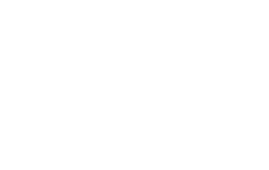7.
1. Expand (1-2x)6 up to term in x3. (2marks)
2. Use the expansion above to evaluate (1.02)6 correct to 4 decimal places. (2marks)
8. Find the length of DP in the figure. (3marks)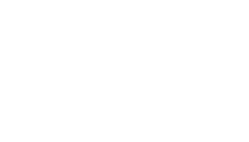9. Given the matrix has no inverse, find the value of x. (2marks)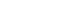10. Without using a calculator solve √252+√72   leaving your answer in the form a√(b+c) where                                                 √(32+√28
a,b and c are integers. (4marks)
11. Find the radius as the center of a circle whose equation is
3x2 + 3y2 + 18y -12x – 9=0 (3marks)
12. A new laptop depreciates at 8% per annum in the first year and 12% per year in the second year. If its value at the end of the second year was sh.121,440, calculate its original value. (3marks)
13. Given that C varies partly as A and partly as the square of A and that C=20 when A=2 and C=21 when A=3,determine the value of C when A=4. (3marks)
14. The size of an interior angle of a regular polygon is 6 ½ times that of its exterior angle determine the number of sides of the polygon. (3 marks)
15. Given that the ration x:y=2:3 find the ratio (5x-2y):(x+y). (3marks)
16. Kiprono buys tea costing sh 112 per kilogram and sh.132 per kilogram and mixes them, then sells the mixture at sh.150 per kilogram .If he is making a profit of 25% in each kilogram of the mixture ,determine the ratio in which he mixes the tea. (4marks)

1. Mrs.Langat ,primary school head teacher earns a basic salary of sh.38,300 house allowance of sh.12,000 and radical ,allowance of sh.3,600 every month. She claims a family relief of sh.1172 and insurance relief of 10% of the premium paid. Using tax rates table below.
 Taxable income£(p.a) Tax(Ksh/£) 1-8800 2 8801-16800 3 16801-24800 5 24801 - 36800 7 36801-48800 9 over 48800 10

1. Calculate Mrs.Langats annual taxable income in Kenya pound per annum. (2marks)
2. Tax due evenly month from Mrs.Langat. (4marks)
3. If the following deductions are made every month from her salary,
w.c p.s 2 % of basic salary
sacco van repayment of sh.14,200
Calculate:
1. the total deductions. (2marks)
2. Her net pay for very month. (2marks)
2. A bag contains 5 red balls, 3 blue balls and 4 yellow balls. Two balls are drawn at random one after the other without replacement.
1. Draw a tree diagram to illustrate his pickings. (2marks)
2. Calculate the probability that
1. Both balls picked were red. (2marks)
2. Both balls picked were of the same colour. (3marks)
3. There was no red ball from the two balls picked. (3marks)
→             →                              →
3. In the triangle given below OP= p and OQ = q.R is a point on PQ  such that PR:RQ = 1 : 3 and that 5OS= 2OQ.PS and OR intersect at T.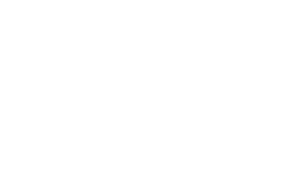1. Express in terms of p and q.
1. OS→ 1mk
2. PQ→ 1mk
2. Given that OT=hOR and PT = kPS, determine the values of h and k. (6marks)
4. In the figure below,P,Q,R and S are points on the circle centre O. PRT and USTV are straight lines.Line UV is a tangent to the circle at S. <RST= 500 and <RTV=1500.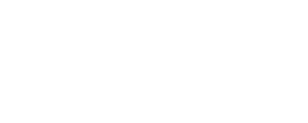1. Calculate the size of
1. < ORS (2marks)
2. <USP (1mark)
3. < PQR (2marks)
2. Given that RT=7cm and ST=9cm,calculate to 3 significant figures
1. Length of the line PR (2marks)
2. The radius of the circle. (3marks)
5. The product of the first three terms of geometric progression is 64.If the first term is a and the common ratio is r;
1. Express r in terms of a (3marks)
2. Given that the sum of the three terms is 14
1. Find the values of a and r ,hence write down two possible sequences each upto 4th term. (5marks)
2. Find the sum of the first 5 terms of each sequences. (2marks)
6. A water vendor has a tank of capacity18,9000 litres.The tank is being filled with water from two pipes A and B which are closed immediately when the tank is full.Water flows at the rate 150,000cm3/minute through pipe B.
1. If the tank is empty ,and the two pipes are opened at the same time,calculate the time it takes to fill the tank. (5marks)
2. On a certain day the vendor opened the two pipes A and B to fill the empty tank.After 25 minutes he opened the outlet tap to supply water to his customer at average rate of 20 litres per minute. Calculate the time it took to fill the tank on that day. (5marks)
7. Three variables p, q and r are such that p varies directly as q and inversely as the square of r.
1. When p=9, q12 and r = 2.
Find p when q= 15 and r =5 (4marks)
2. Express q in terms of p and r. (1mark)
3. If p is increased by 10% and r is decreased by 10%, find;
1. A simplified expression for the change in q in terms of p and r(3marks)
2. The percentage change in q. (2marks)
8. The table below shows some values of the curve y = 2cos x and y= 3 sin x.
1. Complete the table for values y=2cosx and y=3 sin x, correct to 1 decimal places. (3marks)
 x 0 30° 60° 90° 120° 150° 180° 210° 240° 270° 300° 330° 360° y=2cos x 2 1 0 -1.7 -1.7 -1 1 1.7 2 y=3sn x 0 1.5 3 2.6 -2.6 -1.5 0
On the grid provided draw the graphs of y=2 cos x and y= 3sin x for 0° ≤ x ≤ 360° on the same axis. (5marks)
2. Use the graph to find the values of x when 2cos x- 3sin x=0. (2marks)
3. Use the graph to find the values of y when 2 cos x = 3sin x. (1mark)

MARKING SCHEME

SECTION I

1. Use logarithms to evaluate.(3 marks)
4.497 x √0.3673
1- cos 81.53°
2. The sum of the fifth and sixth term of an AP is 30.If the third term is 5.Find the first term.(3marks)
arithmetic progression formular: an=a + (n-1)d
first term a
2nd term: a+d
3rd term: a+2d
4th term: a+3d
5th term: a+4d
6th term: a+5d
(a+4d)+(a+5d)=30
2a+9d=30
(a+2d=5)x2
2a+9d=30
-2a+4d=10
5d=20
d=4
a+2(4)=5
a+8=5
a=5-8=-3
1st term = -3

3. Make K the subject of the formula and simplify.(3marks)
t= 2y+1
√2ky+k

t2= (2y x 1)2
2yk+k

t2(2ky +k)= (2y + 1)2
2ky + k= (2y + 1)2   =k(2y + 1)=(2y+1)2/t 2
t2
k=2y+1
t
2

4. Solve for x
2-(log x)2=3 log x (3marks)
let log x be a
2+a2 =3a
a2 -3a+2=0
a2-2a –a +2=0
a(a-2)-1(1-2)=0 m1
a=1 a=2.
Log x=1 log x=2
X=10 x=100

5. The sides of a triangle were measured and recorded as 4cm,6.2cm and 9.50cm.Calculate the percentage error in its perimeter correct to 2 decimal places. (3marks)
A.E =(4.5 + 6.25 + 9.505)-(3.5 + 6.15 + 9.495)
2
=20.255-19.145=1.11
2             2
=0.555
% error=0.555 x100
19.7
=2.32%

6. The figure below shows a triangle PQR in which PQ=8cm and angle QPR=1000 and angle PQR=350.Calculate to 2 decimal places the length of QR and hence the area of triangle PQR to2 decimal places. (3marks)QR    =     8
Sin 100     sin 35º
QR=8 sin 100º= 13.74cm
Sin 35o
Area = ½x13.74 x 8 sin 45º

=38.86cm2

7.
1. Expand (1-2x)6 up to term in x3. (2marks)
1+ 6(2x)1 + 15 (2x)2 + 20(2x)3
1+12x + 60x2 +160 x3

2. Use the expansion above to evaluate (1.02)6 correct to 4 decimal places. (2marks)
1-2x = 0.98
-2x=0.98-1=-0.02
or 1-0.98=2x
2x=0.02
x=0.01
1+12(0.01 ) + 60(0.01)2+160 (0.01)3
1+ 0.12+0.006 + 0.00016
=1.1262(4 d.p)

8. Find the length of DP in the figure. (3marks)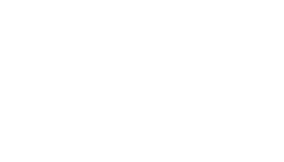AP x BP = CP x DP
9 x 3 = ( 8 + X)X
X2 +8X -27 =0
X= -8 ± √(64+108)
2
=-8 ±√172
2

=-8±13.11
2
=2.557

9. Given the matrix has no inverse, find the value of x. (2marks)(5-x)4 -6x=0
20-4x – 6x =0
20-10x=10
20=10x
X=2

10. Without using a calculator solve √252+√72   leaving your answer in the form a√(b+c) where                                                 √(32+√28
a,b and c are integers. (4marks)
6√7+6√2 = 3√7+√2
4√2+2√7     2√2+√7

3√7+3√2(2√2-√7)
(2√2+√7)(2√2-√7)

6√14 - 2+12-3√14
8-7

3√14 -9

11. Find the radius as the center of a circle whose equation is
3x2 + 3y2 + 18y -12x – 9=0 (3marks)
X2 + y2 – 4x+6y-3=0
X2-4x+4 + y2+6y +9=3+4+9
(x-2)2 +(y+3)2=16

12. A new laptop depreciates at 8% per annum in the first year and 12% per year in the second year. If its value at the end of the second year was sh.121,440, calculate its original value. (3marks)
12. 0.92x x 0.88x = 121,440
X90.92 x 0.88=   121,440
0.92x0.88
X=sh.150,000

13. Given that C varies partly as A and partly as the square of A and that C=20 when A=2 and C=21 when A=3,determine the value of C when A=4. (3marks)
C=hA+KA2
20=2h+4k
21=3h+9k
K=-3 h=16
C=16a-3a2
64-48
=16

14. The size of an interior angle of a regular polygon is 6 ½ times that of its exterior angle determine the number of sides of the polygon. (3 marks)
X+6 ½ X=180O
X + 13/2 X =180O
2X + 13X =360O
X =24O
360/24=15 Sides

15. Given that the ration x:y=2:3 find the ratio (5x-2y):(x+y). (3marks)
X:y  =  2:3
(5x-2y) :(x-y)
(5(2) – 2(3): (2+3)
(10-6):(5)
4:5

16. Kiprono buys tea costing sh 112 per kilogram and sh.132 per kilogram and mixes them, then sells the mixture at sh.150 per kilogram .If he is making a profit of 25% in each kilogram of the mixture ,determine the ratio in which he mixes the tea. (4marks)
Without profit
Cost =  150  = sh 120
1.25
112x +132 y=120
X+y
112x + 132 y = 120
X+y
112x + 132 y=120 x+120 y
12y = 8x
12 = x
8      y
x:y=3:2

1. Mrs.Langat ,primary school head teacher earns a basic salary of sh.38,300 house allowance of sh.12,000 and radical ,allowance of sh.3,600 every month. She claims a family relief of sh.1172 and insurance relief of 10% of the premium paid. Using tax rates table below.
 Taxable income£(p.a) Tax(Ksh/£) 1-8800 2 8801-16800 3 16801-24800 5 24801 - 36800 7 36801-48800 9 over 48800 10

1. Calculate Mrs.Langats annual taxable income in Kenya pound per annum. (2marks)
• 38300 + 1200 + 3600
53900 x 12 = k£ 32,340 pa
20

2. Tax due evenly month from Mrs.Langat. (4marks)
• 32,340
8800 x2=17600
8000 x 3= 24,000
8000 x 5= 40,000
6540 x 7=   45,780
127,380
Monthly = 127,380 = 10,615
12
Less ……. 1172
9443

3. If the following deductions are made every month from her salary,
w.c p.s 2 % of basic salary
sacco van repayment of sh.14,200
Calculate:
1. the total deductions. (2marks)
• 3/100 x 4600 = 198
= 9,245
total decuctions:(9245+776+4600+4200)=Sh.28,821

2. Her net pay for very month. (2marks)
Net pay = 53900-28821=Sh.25079
2. A bag contains 5 red balls, 3 blue balls and 4 yellow balls. Two balls are drawn at random one after the other without replacement.
1. Draw a tree diagram to illustrate his pickings. (2marks)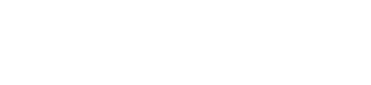2. Calculate the probability that
1. Both balls picked were red. (2marks)
1st picking red: 5/12
2nd picking with no replacement: 4/11
red and red= 5/12 x 4/11= 20/132=5/33

2. Both balls picked were of the same colour. (3marks)
r and r  - 5/33
y and y - 4/12 x 3/11= 12/132 =1/11
b and b - 3/12 x 2/11= 6/132= 1/22
5/33 + 1/11 + 1/22
10+6+3=19
66
66

3. There was no red ball from the two balls picked. (3marks)
No red:
y and y - 4/12 x 3/11= 12/132
y and b - 4/12 x 3/11= 12/132
b and y - 3/12 x 4/11= 12/132
b and b - 3/12 x 2/11= 6/132
=42/132= 7/22

→             →                              →
3. In the triangle given below OP= p and OQ = q.R is a point on PQ  such that PR:RQ = 1 : 3 and that 5OS= 2OQ.PS and OR intersect at T.1. Express in terms of p and q.
1. OS→ 1mk = 2/5q
2. PQ→ 1mk = q-p
3. OR →1mk = 3/4p + 1/4q
2. Given that OT=hOR and PT = kPS, determine the values of h and k. (6marks)
• OT=h (3/4p + 1/4q)
OT=p +k (2/5 q – p)
3/4hp + 1/4hq = 1/4hq=p+2/5kq –kp
3/4h = 1-k h/4=2/5k
3/4h (8k/5) (1-k)
6k/5 = 5-5k
11k=5
K=5/11
11=8/11
PT:T=5/11:6/11
5:6

4. In the figure below,P,Q,R and S are points on the circle centre O. PRT and USTV are straight lines.Line UV is a tangent to the circle at S. <RST= 500 and <RTV=1500.1. Calculate the size of
1. < ORS (2marks)= 40º
2. <USP (1mark)=80º(50 +30)
3. < PQR (2marks)=180º -50º=130º

2. Given that RT=7cm and ST=9cm,calculate to 3 significant figures
1. Length of the line PR (2marks)
• (ST)2=PT:RT
(9)2=PT=7cm
PT=81/7=11.6(3s.f)
11.6 - 7= 4.6

2. The radius of the circle. (3marks)
4.6=    2R
Sin 500
=3.00

5. The product of the first three terms of geometric progression is 64.If the first term is a and the common ratio is r;
1. Express r in terms of a (3marks)
nth= arn-1
a x ar x ar2=64
a3r3=64
r3 =64/a3
r=4/a

2. Given that the sum of the three terms is 14
1. Find the values of a and r ,hence write down two possible sequences each upto 4th term. (5marks)
a + ar + ar2=14
a+a(4/a) + a(4/a)2=14
a+ 4+16/a=14
a2 + 4a + 16 =149 a=2 r=2
a2-10a + 16 =0 a=8 r=½
a2 -8a -2a + 16=0
a(a-8) -2(a-8)=0
a=2 or 8
r=4/8 or 4/2 ½or2
r=½=8,4,2,1,or
r=2=2,4,8,18

2. Find the sum of the first 5 terms of each sequences. (2marks)
S50= a(1-rn) r<1
1-r
(a(rn-1) r)/1-r> 1
(2(ss-1))/2-1 = 62

6. A water vendor has a tank of capacity18,9000 litres.The tank is being filled with water from two pipes A and B which are closed immediately when the tank is full.Water flows at the rate 150,000cm3/minute through pipe B.
1. If the tank is empty ,and the two pipes are opened at the same time,calculate the time it takes to fill the tank. (5marks)
150,000
+120,000
270,000cm3/min
270 c/min
= 18,900= 70min
270

2. On a certain day the vendor opened the two pipes A and B to fill the empty tank.After 25 minutes he opened the outlet tap to supply water to his customer at average rate of 20 litres per minute. Calculate the time it took to fill the tank on that day. (5marks)
270 l/min x 25 =6,750 litres by one
He opened to his custom
18,900- 6750 = 12,150 litres
Rate of filling = 270-20=250
483/5min

7. Three variables p, q and r are such that p varies directly as q and inversely as the square of r.
1. When p=9, q12 and r = 2.
Find p when q= 15 and r =5 (4marks)
• P=kQ/r2
9= 12k/22
k=9/3 =3

P= 3(15)/25
P=1.8

2. Express q in terms of p and r. (1mark)
P=KQ/r2
(pr2)/3=q

3. If p is increased by 10% and r is decreased by 10%, find;
1. A simplified expression for the change in q in terms of p and r(3marks)
2. The percentage change in q. (2marks)
8. The table below shows some values of the curve y = 2cos x and y= 3 sin x.
1. Complete the table for values y=2cosx and y=3 sin x, correct to 1 decimal places. (3marks)
 x 0 30° 60° 90° 120° 150° 180° 210° 240° 270° 300° 330° 360° y=2cos x 2 1 0 -1.7 -1.7 -1 1 1.7 2 y=3sn x 0 1.5 3 2.6 -2.6 -1.5 0
On the grid provided draw the graphs of y=2 cos x and y= 3sin x for 0° ≤ x ≤ 360° on the same axis. (5marks)
2. Use the graph to find the values of x when 2cos x- 3sin x=0. (2marks)
3. Use the graph to find the values of y when 2 cos x = 3sin x. (1mark)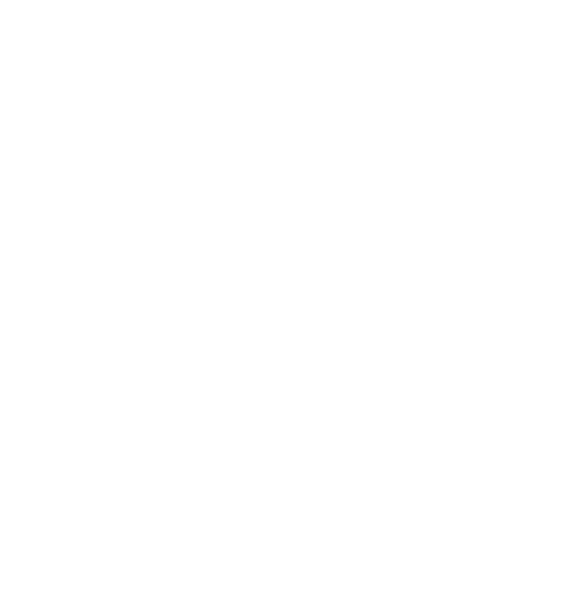• ✔ To read offline at any time.
• ✔ To Print at your convenience
• ✔ Share Easily with Friends / Students